# JavaScript | 思维导图 | 这些高阶函数你都会了吗？#### 0 / 闭包作用域练习题

``````let x = 5;
function fn(x){
return function (y) {
console.log(y + (++x));
}
}
let f = fn(6);
f(7);
fn(8)(9);
f(10);
console.log(x);

△ 结果是？

##### （1）`++i` 和 `i++` 的区别
``````let i = 1;
i++;
console.log(i);

i=1;
i+=1; // i=i+1;
console.log(i);

△ 结果是？

``````let i = '1';
i++;
console.log(i);

i='1';
i+=1; // i=i+1;
console.log(i);

△ 结果是？

`i++/++i` 一定是数学运算，`+N` 也是把N变为数字类型的值

`++i``i++` 的区别：

[相同点]：都是自身基础上累加1

[不同点]：计算和累加的顺序

`++i` 自身先累加1，再根据累加后的结果进行运算

`i++` 先根据原始值进行运算，运算完之后再自身累加1

``````let i = 1;
console.log(5 + i++);
//=> 或者 5+(i++)
// ① 先算 5+i
// ② 再 i++: i=2
console.log(i); //=> 2

i = 1;
console.log(5 + (++i));
// ① 先算 ++i : i=2
// ② 5+i = 7

△ 区别

``````let i = 2;
console.log(2 + (++i) - (i++) + 3 - (i--) + (i--));
console.log(i);

△ 自己算吧

##### （2）图解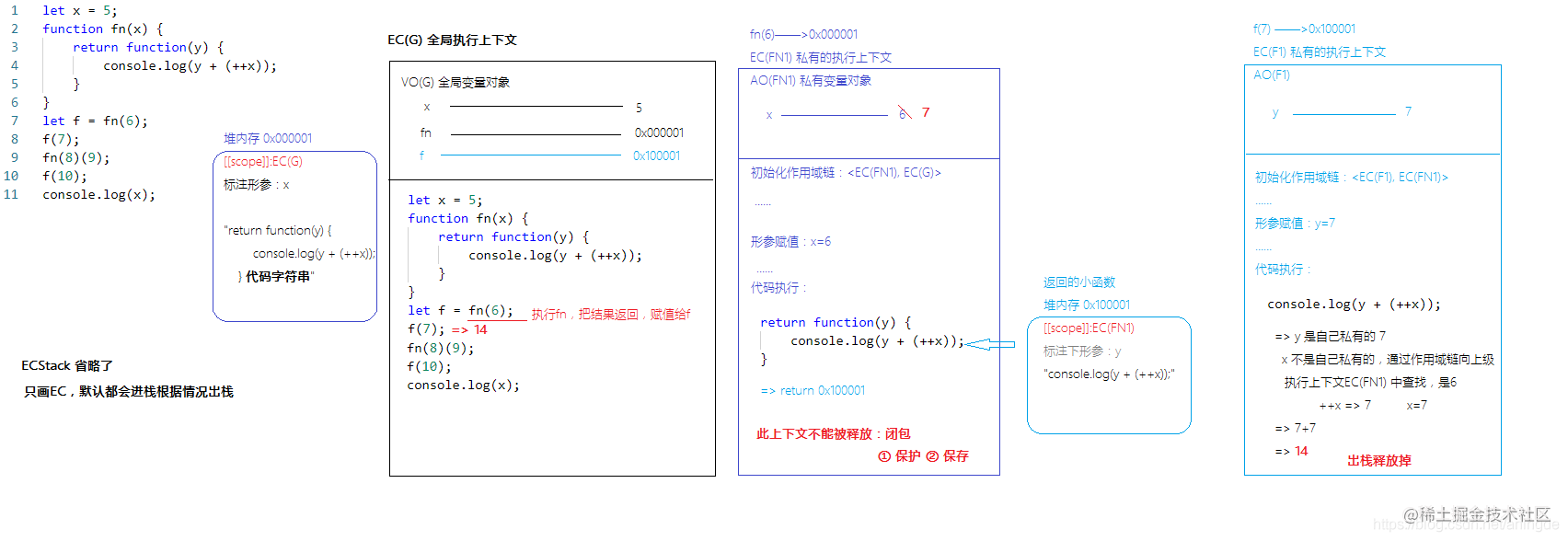△ 图1.1_注意：函数创建和函数执行

`fn(8) ——>0x000001(8)` 执行完后，返回值`0x100002`紧接着会被执行，那么`EC(FN2)`这个私有的执行上下文临时不被释放，当它用完以后才会被释放掉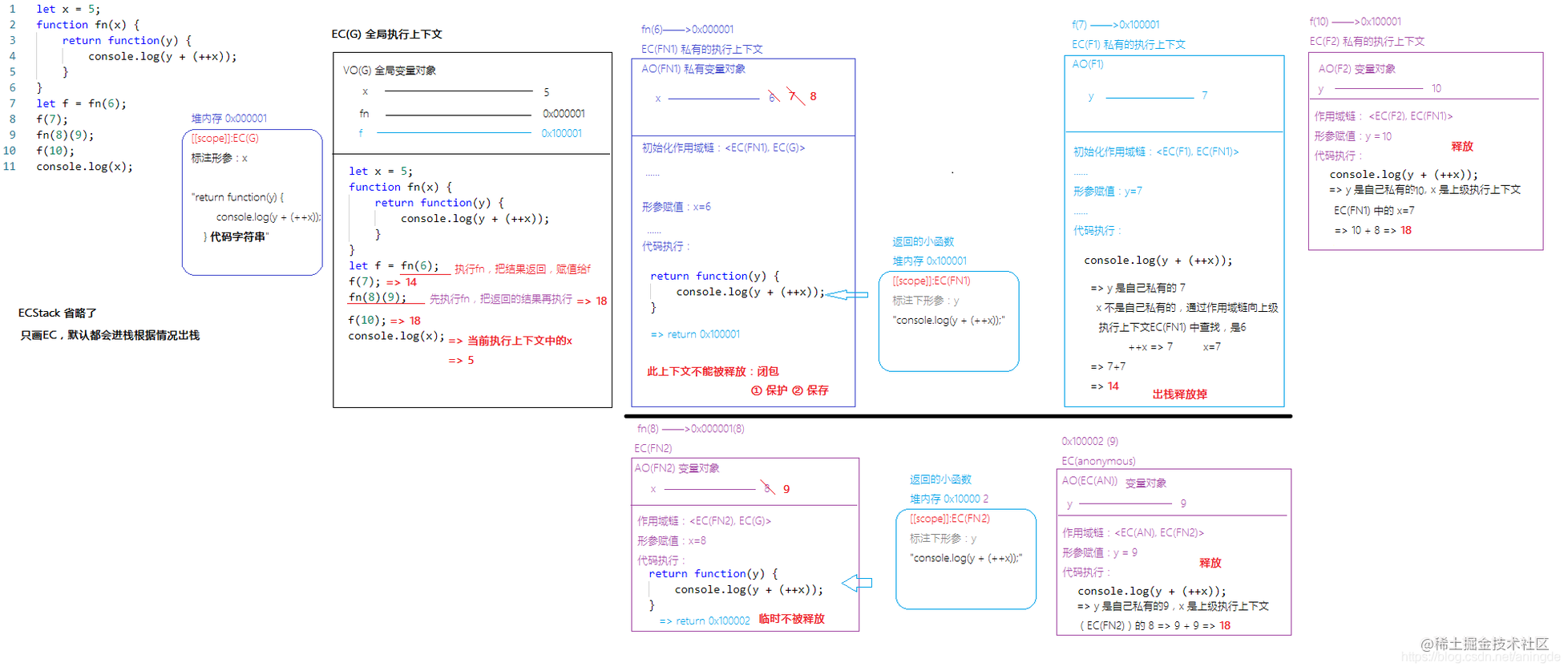△ 图1.2_注意：函数创建和函数执行

GC ：浏览器的垃圾回收机制，内存管理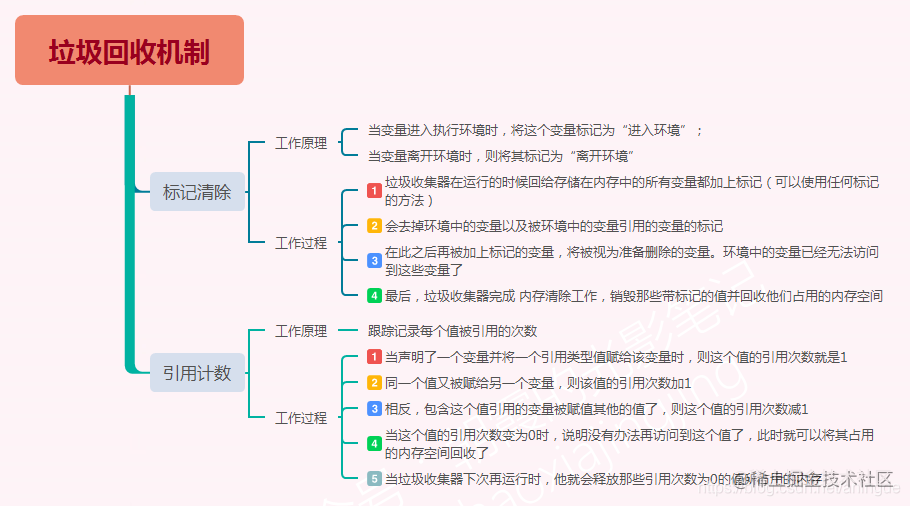###### ①【谷歌】：查找引用

[堆内存]：当前堆内存如果被占用了（指针关联地址了）则不能释放掉；如果没有任何的事物占用这个堆，则浏览器会自动把这个堆内存释放掉

[栈内存]：当前执行上下文中是否有内容（一般是堆内存）被此上下文以外的事物所占用，如果被占用则无法释放（闭包），如果没有被占用则释放掉。其中，EC(G) 是在加载页面时候创建，只有在关闭页面时候才会释放掉

【思考题】总结内存泄漏的情况

###### ③ 手动优化

`null` 是不占用空间的，把占用的事物手动赋值为null，可以实现内存的手动优化

`fn = null` 释放0x000001

`f = null` 释放0x100001 后，EC(FN1) 也会被释放掉

##### （3）重构函数
``````let a=0,
b=0;
function A(a){
A=function(b){
};
}
A(1);
A(2);

△ 函数重构

``````let a = 1,
b = 2;
// 等价于
let a = 1;
let b = 2;
//==============
let a = b =1;
// 等价于
b = 1;
let a = 1;

△ 赋值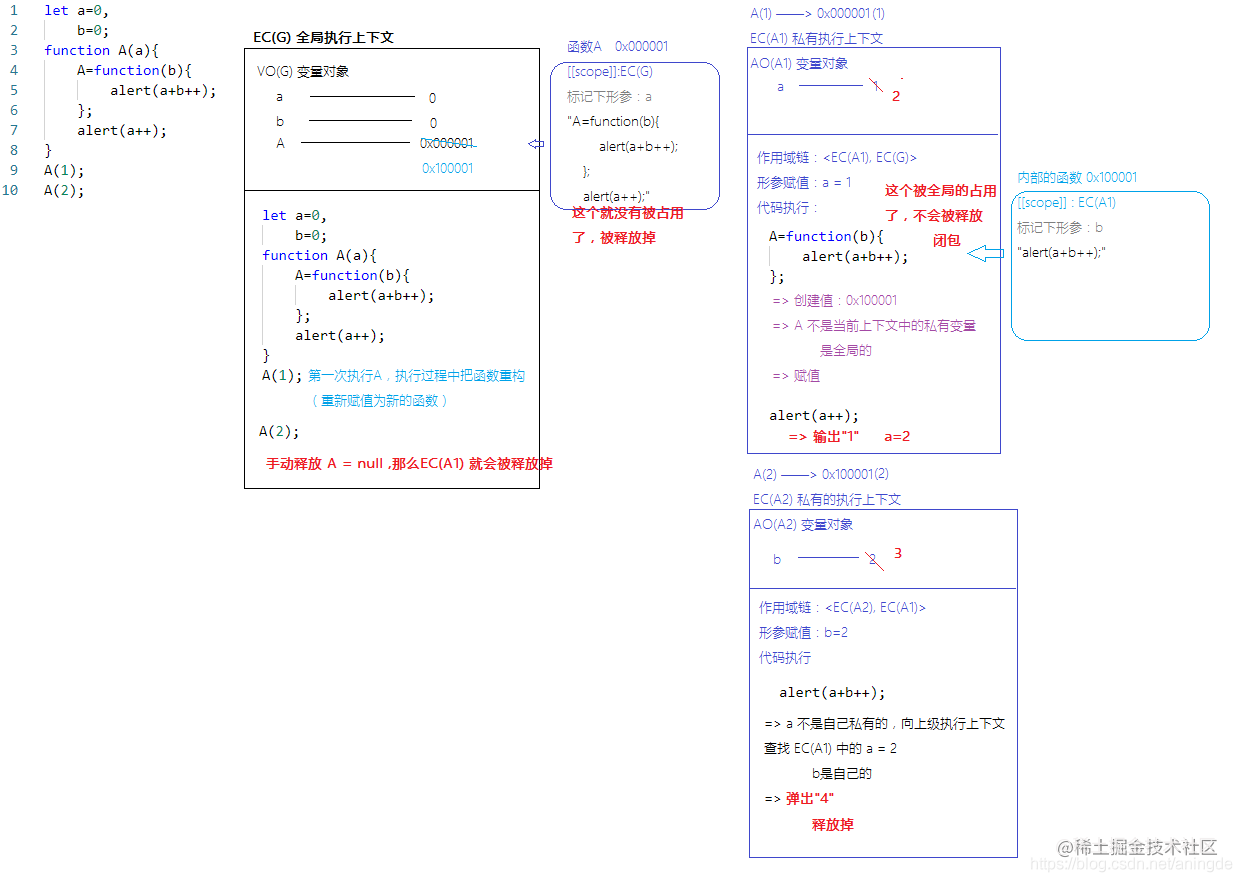△ 图1.3_函数重构图解

#### 1 / this的5种基础情况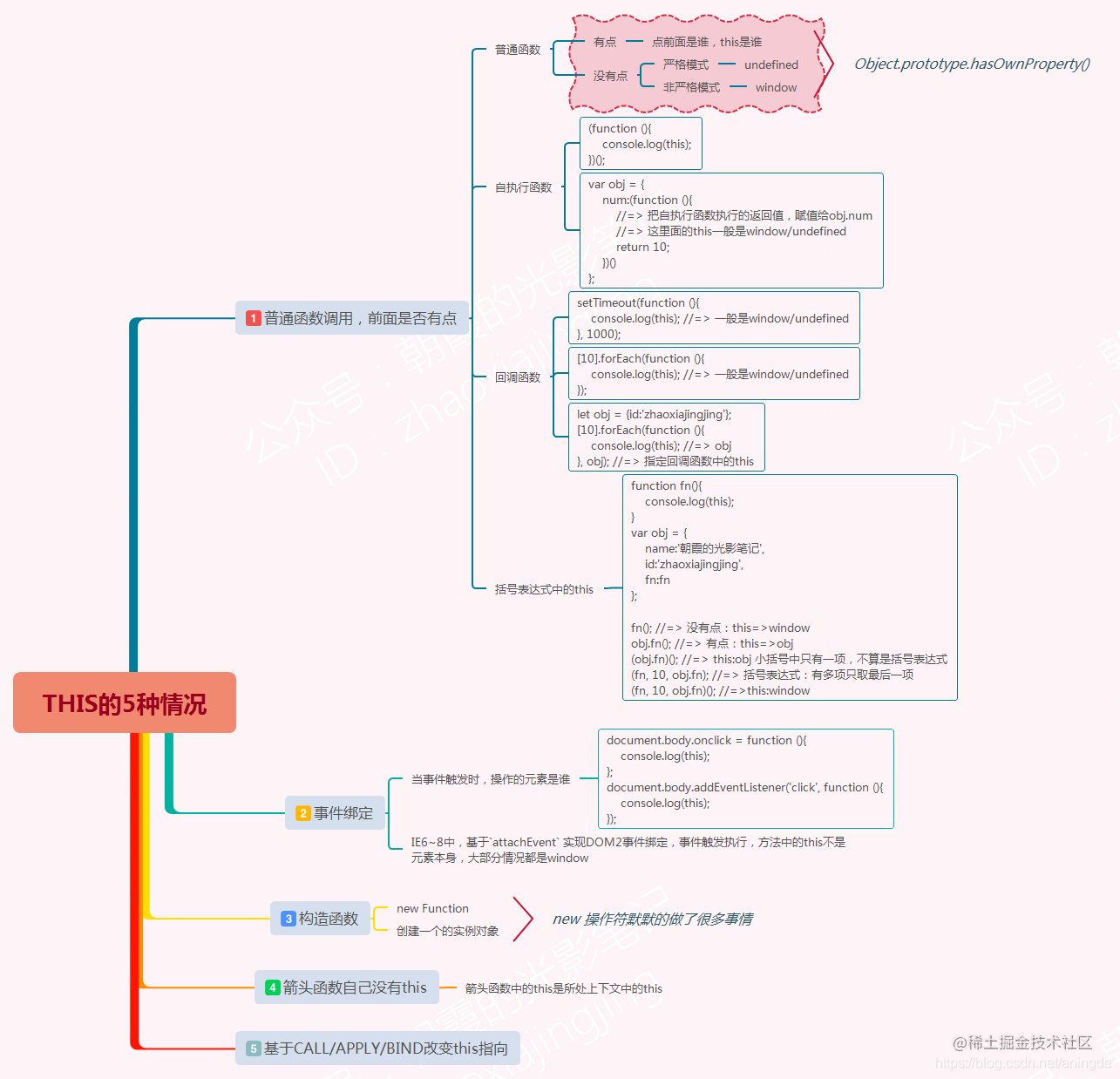`this`是函数的执行主体，不等价于执行上下文/作用域。

`this`就是谁把函数执行了

1、事件绑定

2、普通函数执行

3、构造函数执行

4、箭头函数执行

5、基于call/apply/bind强制改变this

``````"use strict;"
console.log(this); //=> window

△ 非函数中的this

##### （1）事件绑定
``````document.body.onclick = function (){
console.log(this);
};
console.log(this);
});

△ 事件绑定

1、不论是DOM0还是DOM2级事件绑定，给元素E的某个事件行为绑定方法，当事件触发方法执行时，方法中的this是当前元素E本身

2、特殊情况：

（1）IE6~8中，基于`attachEvent` 实现DOM2事件绑定，事件触发执行，方法中的this不是元素本身，大部分情况都是window

（2）基于`call/apply/bind`强制改变了函数中的this，我们也是以强制改变为主

##### （2）普通函数执行
###### ① 普通函数

1、有“点”，“点”前面是谁this就是谁

2、没有“点”，this是window。JS严格模式下是：undefined

``````function fn(){
console.log(this);
}
var obj = {
name:'朝霞的光影笔记',
id:'zhaoxiajingjing',
fn:fn
};

fn(); //=> 没有点：this=>window
obj.fn(); //=> 有点：this=>obj

△ 普通函数的this

`xxx.__proto__.fn()`：方法执行，前面有“点”，this是：`xxx.__proto__`

###### ② 自执行函数

``````(function (){
console.log(this);
})();

△ 自执行函数

``````var obj = {
num:(function (){
//=> 把自执行函数执行的返回值，赋值给obj.num
//=> 这里面的this一般是window/undefined
return 10;
})()
};

△ 自执行函数

###### ③ 回调函数

``````setTimeout(function (){
console.log(this); //=> 一般是window/undefined
}, 1000);

.forEach(function (){
console.log(this); //=> 一般是window/undefined
});

let obj = {id:'zhaoxiajingjing'};
.forEach(function (){
console.log(this); //=> obj
}, obj); //=> 指定回调函数中的this

△ 回调函数

###### ④ 括号表达式中的this很变态
``````function fn(){
console.log(this);
}
var obj = {
name:'朝霞的光影笔记',
id:'zhaoxiajingjing',
fn:fn
};

fn(); //=> 没有点：this=>window
obj.fn(); //=> 有点：this=>obj
(obj.fn)(); //=> this:obj 小括号中只有一项，不算是括号表达式
(fn, 10, obj.fn); //=> 括号表达式：有多项只取最后一项
(fn, 10, obj.fn)(); //=>this:window

△ 括号表达式

##### （3）题目
``````var x = 3,
obj = {x: 5};
obj.fn = (function () {
this.x *= ++x;
return function (y) {
this.x *= (++x)+y;
console.log(x);
}
})();
var fn = obj.fn;
obj.fn(6);
fn(4);
console.log(obj.x, x);

△ 找THIS

`a+=b-c` => a = a+(b-c)

`a*=b-c` => a = a* (b-c)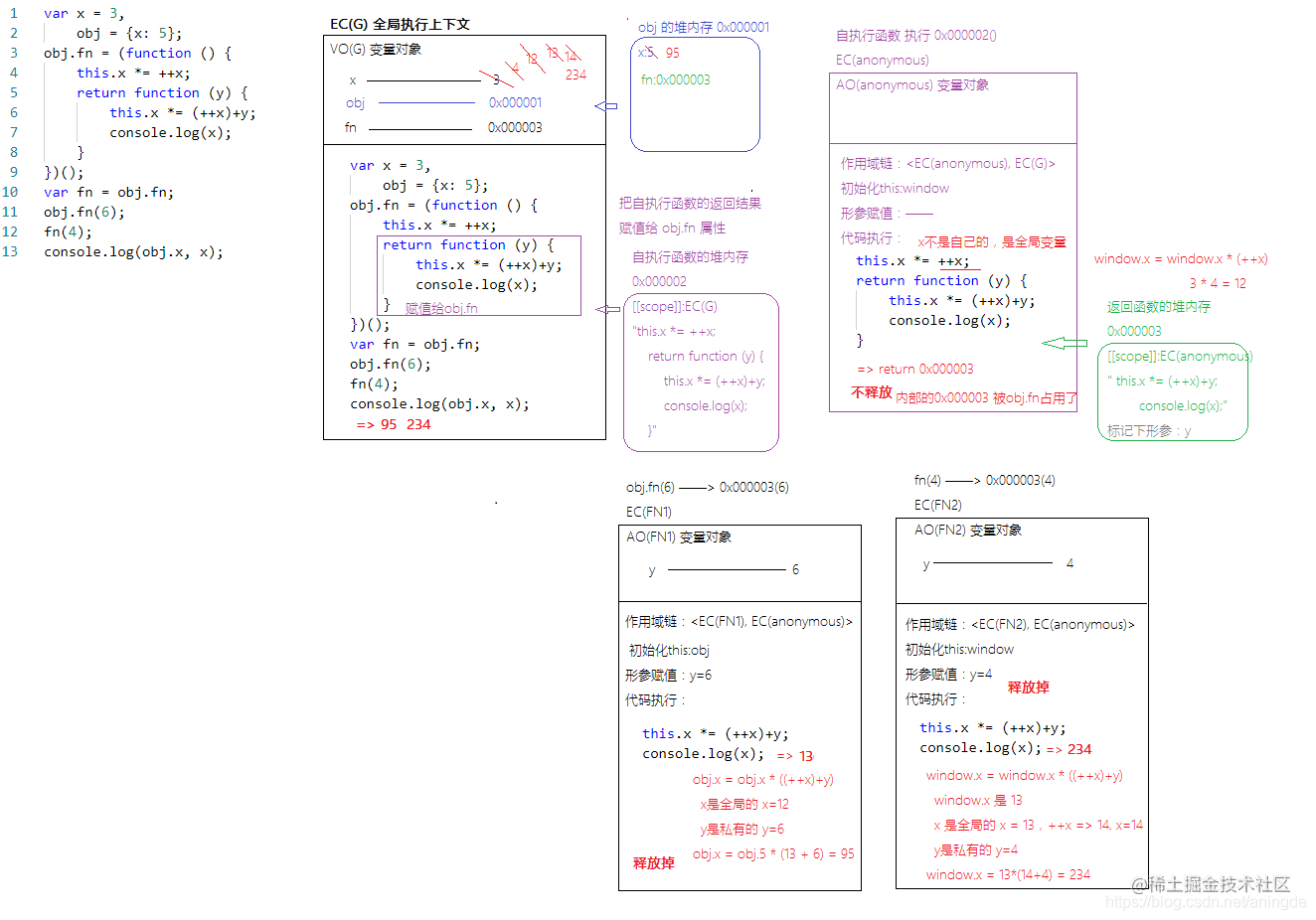△ 图1.4_图解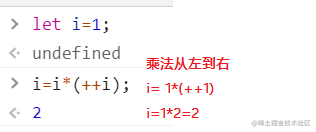△ 图1.5_计算过程

#### 2 / JS高阶编程技巧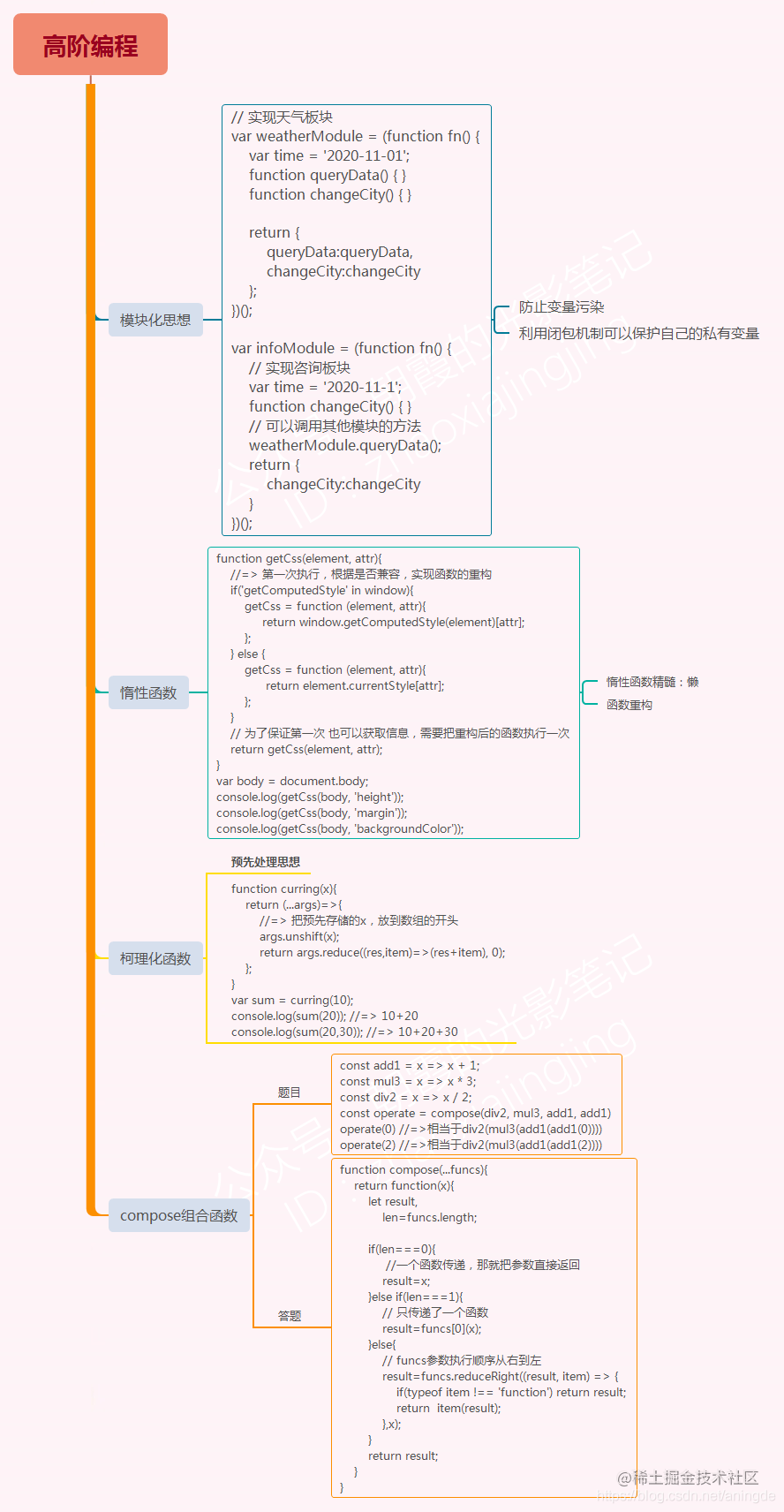JS高阶编程技巧：利用闭包的机制，实现出来的一些高阶编程的方式

1、模块化思想

2、惰性函数

3、柯理化函数

4、compose组合函数

5、高阶组件 React中的

6、……

##### （1）模块化思想

``````// 实现天气板块
var time = '2020-11-01';
function queryData(){
// ...CODE
}
function changeCity(){
// ...CODE
}

// 实现咨询板块
var time = '2020-11-1';
function changeCity(){
// ...CODE
}

△ 很久以前的没有模块化思想之前

###### ① 闭包

``````// 实现天气板块
(function fn() {
var time = '2020-11-01';
function queryData() { }
function changeCity() {  }
})();

(function fn() {
// 实现咨询板块
var time = '2020-11-1';
function changeCity() { }
})();

△ 基于闭包的保护作用

###### ② 某一种方案

``````// 实现天气板块
(function fn() {
var time = '2020-11-01';
function queryData() { }
function changeCity() {  }
window.queryData=queryData;
window.changeCity=changeCity;
})();

(function fn() {
// 实现咨询板块
var time = '2020-11-1';
function changeCity() { }
window.changeCity=changeCity;
})();

△ 挂在window上

###### ③ 再一种方案

``````let obj1 = {
name:'朝霞的光影笔记',
id:'zhaoxiajingjing',
show:function(){}
};
let obj2 = {
name:'zxjj',
show:function (){}
};

△ 对象

###### ④ 进阶一下

``````// 实现天气板块
var weatherModule = (function fn() {
var time = '2020-11-01';
function queryData() { }
function changeCity() { }

return {
queryData:queryData,
changeCity:changeCity
};
})();

var infoModule = (function fn() {
// 实现咨询板块
var time = '2020-11-1';
function changeCity() { }
// 可以调用其他模块的方法
weatherModule.queryData();
return {
changeCity:changeCity
}
})();

△ 单例模式

##### （2）惰性函数

`window.getComputedStyle(document.body)`获取当前元素经过浏览器计算的样式，返回样式对象

###### ① 一开始这样写
``````function getCss(element, attr){
if('getComputedStyle' in window){
return window.getComputedStyle(element)[attr];
}
return element.currentStyle[attr];
}
var body = document.body;
console.log(getCss(body, 'height'));
console.log(getCss(body, 'margin'));
console.log(getCss(body, 'backgroundColor'));

△ 获取样式

【优化思想】：第一次执行`getCss`我们就知道是否兼容了，第二次及以后再次调用`getCss`时，则不想再处理兼容的容错处理了，这就是“惰性思想”【就是“懒”，干一次可以搞定的，绝对不去做第二次了

###### ② 优化一下

``````var flag = 'getComputedStyle' in window;

function getCss(element, attr){
if(flag){
return window.getComputedStyle(element)[attr];
}
return element.currentStyle[attr];
}
var body = document.body;
console.log(getCss(body, 'height'));
console.log(getCss(body, 'margin'));
console.log(getCss(body, 'backgroundColor'));

△ 优化一下

###### ③ 惰性思想

``````function getCss(element, attr){
//=> 第一次执行，根据是否兼容，实现函数的重构
if('getComputedStyle' in window){
getCss = function (element, attr){
return window.getComputedStyle(element)[attr];
};
} else {
getCss = function (element, attr){
return element.currentStyle[attr];
};
}
// 为了保证第一次 也可以获取信息，需要把重构后的函数执行一次
return getCss(element, attr);
}
var body = document.body;
console.log(getCss(body, 'height'));
console.log(getCss(body, 'margin'));
console.log(getCss(body, 'backgroundColor'));

△ 惰性函数+重构函数

##### （3）柯理化函数

``````//=> x 是预先存储的值
function curring(x){}
var sum = curring(10);
console.log(sum(20)); //=> 10+20
console.log(sum(20,30)); //=> 10+20+30

△ 请实现柯理化函数

###### ① 把类数组转换为数组：
``````let arg = {0:10, 1:20, length:2};
let arr = [...arg];
arr = Array.from(arg);
arr = [].slice.call(arg);

△ 类数组转为数组

###### ② 数组求和

1、for循环/forEach

2、`eval([10,20,30].join('+'))`

3、`[10,20,30].reduce()`

###### ③ 数组的reduce方法

API：developer.mozilla.org/zh-CN/docs/…

`arr.reduce(callback()[, initialValue])`

`callback(accumulator, currentValue[, index[, array]])`

``````let arr = [10,20,30,40];
let res = arr.reduce(function (result, item, index){
console.log(result, item, index);
});

△ reduce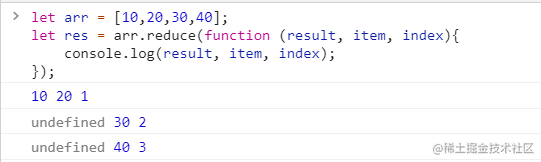△ 图1.6_reduce执行

1、initialValue 初始值不传递，result 默认初始值是数组的第一项，reduce是从数字第二项开始遍历的

2、每遍历数组中的一项，回调函数被触发执行一次

① result 存储的是上一次回调函数返回的结果。除了第一次是初始值或者数字第一项

② item 当前遍历这一项

③ index 当前遍历这一项的索引

``````let arr = [10,20,30,40];
let res = arr.reduce(function (result, item, index){
console.log(result, item, index);
return item + result;
});
console.log(res); //=> 100

△ arr.reduce

``````let arr = [10,20,30,40];
let res = arr.reduce(function (result, item, index){
console.log(result, item, index);
}, 0 );

△ reduce 传递初始值了

arr.reduce 传递了initialValue了，则result 的第一次结果就是初始值，item从数组第一项开始遍历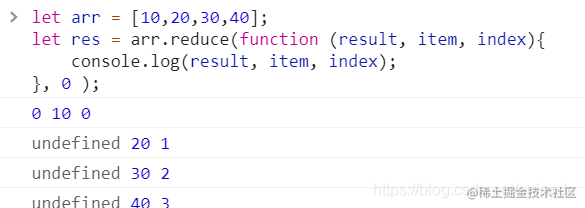△ 图1.7_reduce执行

``````Array.prototype.reduce = function reduce(callback, initialValue){
let self = this,
i = 0;    //=> THIS:arr

if(typeof callback !== 'function') throw new TypeError('callback must be a function');

if(typeof initialValue === "undefined"){
initialValue = self;
i = 1;
}
// 迭代数组每一项
for(; i < self.length; i++){
var item = self[i],
index = i;
initialValue = callback(initialValue, item, index, self);
}
return initialValue;
};

△ 自己手写reduce

###### ④ 柯理化函数
``````function curring(x){
return (...args)=>{
//=> 把预先存储的x，放到数组的开头
args.unshift(x);
return args.reduce((res,item)=>(res+item), 0);
};
}
var sum = curring(10);
console.log(sum(20)); //=> 10+20
console.log(sum(20,30)); //=> 10+20+30

△ 柯理化函数

##### （4）compose 组合函数
###### ① 题目描述

``````const add1 = x => x + 1;
const mul3 = x => x * 3;
const div2 = x => x / 2;

△ 函数组合

``````const operate = compose(div2, mul3, add1, add1)

△ 可读性较好

​ 简而言之：compose可以把类似于`f(g(h(x)))`这种写法简化成`compose(f, g, h)(x)`，请你完成 compose函数的编写

###### ② 答题
``````function compose(...funcs){
return function(x){
let result,
len=funcs.length;

if(len===0){
//一个函数传递，那就把参数直接返回
result=x;
}else if(len===1){
// 只传递了一个函数
result=funcs(x);
}else{
// funcs参数执行顺序从右到左
result=funcs.reduceRight((result, item) => {
if(typeof item !== 'function') return result;
return  item(result);
},x);
}
return result;
}
}

△ 实现compose组合函数

#### 3 / 思考题

1、内存泄漏

2、严格模式和非严格模式区别API：developer.mozilla.org/zh-CN/docs/…

3、分析redux 中的compose 方法的实现逻辑

``````function compose(...funcs) {
if (funcs.length === 0) {
return arg => arg
}

if (funcs.length === 1) {
return funcs
}

return funcs.reduce((a, b) => (...args) => a(b(...args)))
}

△ redux中的compose实现

- end -